### 5. BIAS AND HIERARCHICAL CLUSTERING

The biggest stumbling-block for attempts to confront theories of cosmological structure formation with observations of galaxy clustering is the uncertain and possibly biased relationship between galaxies and the distribution of gravitating matter. The idea that galaxy formation might be biased goes back to the realization by Kaiser (1984) that the reason Abell clusters display stronger correlations than galaxies at a given separation is that these objects are selected to be particularly dense concentrations of matter. As such, they are very rare events, occurring in the tail of the distribution function of density fluctuations. Under such conditions a ``high-peak'' bias prevails: rare high peaks are much more strongly clustered than more typical fluctuations (Bardeen et al. 1986). If the properties of a galaxy (its morphology, color, luminosity) are influenced by the density of its parent halo, for example, then differently-selected galaxies are expected to a different bias (e.g. Dekel & Rees 1987). Observations show that different kinds of galaxy do cluster in different ways (e.g. Loveday et al. 1995; Hermit et al. 1996).

In local bias models, the propensity of a galaxy to form at a point where the total (local) density of matter isis taken to be some function f () (Coles 1993, hereafter C93; Fry & Gaztanaga 1993, hereafter FG93). It is possible to place stringent constraints on the effect this kind of bias can have on galaxy clustering statistics without making any particular assumption about the form of f. In this Letter, we describe the results of a different approach to local bias models that exploits new results from the theory of hierarchical clustering in order to place stronger constraints on what a local bias can do to galaxy clustering. We leave the technical details to Munshi et al. (1999a, b) and Bernardeau & Schaeffer (1999); here we shall simply motivate and present the results and explain their importance in a wider context.

The fact that Newtonian gravity is scale-free suggests that the N-point correlation functions of self-gravitating particles,N, evolved into the large-fluctuation regime by the action of gravity, should obey a scaling relation of the form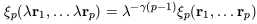(39)

when the elements of a structure are scaled by a factor(e.g. Balian & Schaeffer 1989). Observations offer some support for such an idea, in that the observed two-point correlation function(r) of galaxies is reasonably well represented by a power law over a large range of length scales,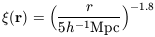(40)

(Groth & Peebles 1977; Davis & Peebles 1977) for r between, say, 100h-1 kpc and 10h-1 Mpc. The observed three point function,3, is well-established to have a hierarchical form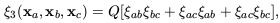(41)

whereab =(xa, xb), etc, and Q is a constant (Davis & Peebles 1977; Groth & Peebles 1977). The four-point correlation function can be expressed as a combination of graphs with two different topologies - ``snake'' and ``star'' - with corresponding (constant) amplitudes Ra and Rb respectively:(42)

(e.g. Fry & Peebles 1978; Fry 1984).

It is natural to guess that all p-point correlation functions can be expressed as a sum over all possible p-tree graphs with (in general) different amplitudes Qp,for each tree diagram topology. If it is further assumed that there is no dependence of these amplitudes upon the shape of the diagram, rather than its topology, the correlation functions should obey the following relation: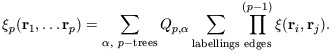(43)

To go further it is necessary to find a way of calculating Qp. One possibility, which appears remarkably successful when compared with numerical experiments (Munshi et al. 1999b; Bernardeau & Schaeffer 1999), is to calculate the amplitude for a given graph by simply assigning a weight to each vertex of the diagram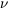n, where n is the order of the vertex (the number of lines that come out of it), regardless of the topology of the diagram in which it occurs. In this case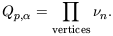(44)

Averages of higher-order correlation functions can be defined as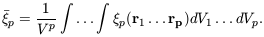(45)

Higher-order statistical properties of galaxy counts are often described in terms of the scaling parameters Sp constructed from the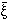p via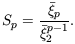(46)

It is a consequence of the particular class of hierarchical clustering models defined by equations (5) & (6) that all the Sp should be constant, independent of scale.

Using a generating function technique [Bernardeau & Schaeffer 1992] it is possible to derive a series expansion for the m-point count probability distribution function of the objects Pm(N1, .... Nm) (the joint probability of finding Ni objects in the i-th cell, where i runs from 1 to m) from then. The hierarchical model outlined above is therefore statistically complete. In principle, therefore, any statistical property of the evolved distribution of matter can be calculated just as it can for a Gaussian random field. This allows us to extend various results concerning the effects of biasing on the initial conditions into the nonlinear regime in a more elegant way than is possible using other approaches to hierarchical clustering.

For example, let us consider the joint probability P2(N1, N2) for two cells to contain N1 and N2 particles respectively. Using the generating-function approach outlined above, it is quite easy to show that, at lowest order,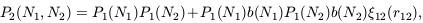(47)

where the P1(Ni) are the individual count probabilities of each volume separately and12 is the underlying mass correlation function. The function b(Ni) we have introduced in (9) depends on the set ofn appearing in equation (6); its precise form does not matter in this context, but the structure of equation (9) is very useful. We can use (9) to define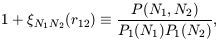(48)

whereN1N2(r12) is the cross-correlation of ``cells'' of occupancy N1 and N2 respectively. From this definition and equation (9) it follows that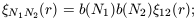(49)

we have dropped the subscripts on r for clarity from now on. From (11) we can obtain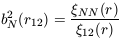(50)

for the special case where N1 = N2 = N which can be identified with the usual definition of the bias parameter associated with the correlations among a given set of objectsobj(r) = b2objmass(r). Moreover, note that at this order (which is valid on large scales), the correlation bias defined by equation (11) factorizes into contributions bNi from each individual cell (Bernardeau 1996; Munshi et al. 1999b).

Coles (1993) proved, under weak conditions on the form of a local bias f () as discussed in the introduction, that the large-scale biased correlation function would generally have a leading order term proportional to12(r12). In other words, one cannot change the large-scale slope of the correlation function of locally-biased galaxies with respect to that of the mass. This ``theorem'' was proved for bias applied to Gaussian fluctuations only and therefore does not obviously apply to galaxy clustering, since even on large scales deviations from Gaussian behaviour are significant. It also has a more minor loophole, which is that for certain peculiar forms of f the leading order term is proportional to122, which falls off more sharply than12 on large scales.

Steps towards the plugging of this gap began with FG93 who used an expansion of f in powers ofand weakly non-linear (perturbative) calculations of12(r) to explore the statistical consequences of biasing in more realistic (i.e. non-Gaussian) fields. Based largely on these arguments, Scherrer & Weinberg (1998), hereafter SW98, confirmed the validity of the C93 result in the non-linear regime, and also showed explicitly that non-linear evolution always guarantees the existence of a linear leading-order term regardless of f, thus plugging the small gap in the original C93 argument. These works have a similar motivation the approach I am discussing here, and also exploit hierarchical scaling arguments of the type discussed above en route to their conclusions. What is different about the approach we have used in this paper is that the somewhat cumbersome simultaneous expansion of f and12 used by SW98 is not required in this calculation. The generating functions to proceed directly to the joint probability (9), while SW98 have to perform a complicated sum over moments of a bivariate distribution. The factorization of the probability distribution (9) is also a stronger result than that presented by SW98, in that it leads almost trivially to the C93 ``theorem'' but also generalizes to higher-order correlations than the two-point case under discussion here.

Note that the density of a cell of given volume is simply proportional to its occupation number N. The factorizability of the dependence ofN1N2(r12) upon b(N1) and b(N2) in (11) means that applying a local bias f () boils down to applying some bias function F(N) = f[b(N)] to each cell. Integrating over all N thus leads directly to the same conclusion as C93, i.e. that the large-scale(r) of locally-biased objects is proportional to the underlying matter correlation function. This has also been confirmed by numerically using N-body experiments (Mann et al. 1998; Narayanan et al. 1998).

In hierarchical models, galaxy formation involves the following three stages:

1. the formation of a dark matter halo;

2. the settling of gas into the halo potential;

3. the cooling and fragmentation of this gas into stars.

Rather than attempting to model these stages in one go by a simple function f of the underlying density field it is interesting to see how each of these selections might influence the resulting statistical properties. Bardeen et al. (1986), inspired by Kaiser (1984), pioneered this approach by calculating detailed statistical properties of high-density regions in Gaussian fluctuations fields. Mo & White (1996) and Mo et al. (1997) went further along this road by using an extension of the Press-Schechter (1974) theory to calculate the correlation bias of halos, thus making an attempt to correct for the dynamical evolution absent in the Bardeen et al. approach. The extended Press-Schechter approach seem to be in good agreement with numerical simulations, except for small halo masses (Jing 1998). It forms the basis of many models for halo bias in the subsequent literature (e.g. Moscardini et al. 1998; Tegmark & Peebles 1998).

The hierarchical models furnish an elegant extension of this work that incorporates both density-selection and non-linear dynamics in an alternative to the Mo & White (1996) approach. We exploit the properties of equation (47) to construct the correlation function of volumes where the occupation number exceeds some critical value. For very high occupations these volumes should be in good correspondence with collapsed objects.

The way of proceeding is to construct a tree graph for all the points in both volumes. One then has to re-partition the elements of this graph into internal lines (representing the correlations within each cell) and external lines (representing inter-cell correlations). Using this approach the distribution of high-density regions in a field whose correlations are given by eq. (5) can be shown to be itself described by a hierarchical model, but one in which the vertex weights, say Mn, are different from the underlying weightsn (Bernardeau & Schaeffer 1992, 1999; Munshi et al. 1999a, b).

First note that a density threshold is in fact a form of local bias, so the effects of halo bias are governed by the same strictures as described in the previous section. Many of the other statistical properties of the distribution of dense regions can be reduced to a dependence on a scaling parameter x, where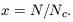(51)

In this definition Nc =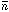2, whereis the mean number of objects in the cell and2 is defined by eq. (45) with p = 2. The scaling parameters Sp can be calculated as functions of x, but are generally rather messy (Munshi et al. 1999a). The most interesting limit when x >> 1 is, however, rather simple. This is because the vertex weights describing the distribution of halos depend only on then and this dependence cancels in the ratio (46). In this regime,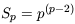(52)

for all possible hierarchical models. The reader is referred to Munshi et al. (1999a) for details. This result is also obtained in the corresponding limit for very massive halos by Mo et al. (1997). The agreement between these two very different calculations supports the inference that this is a robust prediction for the bias inherent in dense regions of a distribution of objects undergoing gravity-driven hierarchical clustering.

The main purpose of this lecture has been to discuss recent developments in the theory of gravitational-driven hierarchical clustering. The model described in equations (5) & (6) provides a statistically-complete prescription for a density field that has undergone hierarchical clustering. This allows us to improve considerably upon biasing arguments based on an underlying Gaussian field.

These methods allow a simpler proof of the result obtained by SW98 that strong non-linear evolution does not invalidate the local bias theorem of C93. They also imply that the effect of bias on a hierarchical density field is factorizable. A special case of this is the bias induced by selecting regions above a density threshold. The separability of bias predicted in this kind of model could be put to the test if a population of objects could be found whose observed characteristics (luminosity, morphology, etc.) were known to be in one-to-one correspondence with the halo mass. Likewise, the generic prediction of higher-order correlation behaviour described by the behaviour of Sp in equation (46) can also be used to construct a test of this particular form of bias.

Referring to the three stages of galaxy formation described in § 4, analytic theory has now developed to the point where it is fairly convincing on (1) the formation of halos. Numerical experiments are beginning now to handle (2) the behaviour of the gas component (Blanton et al. 1998, 1999). But it is unlikely that much will be learned about (3) by theoretical arguments in the near future as the physics involved is poorly understood (though see Benson et al. 1999). Arguments have already been advanced to suggest that bias might not be a deterministic function of, perhaps because of stochastic or other hidden effects (Dekel & Lahav 1998; Tegmark & Bromley 1999). It also remains possible that large-scale non-local bias might be induced by environmental effects (Babul & White 1991; Bower et al. 1993).

Before adopting these more complex models, however, it is important to exclude the simplest ones, or at least deal with that part of the bias that is attributable to known physics. At this stage this means that the `minimal' bias model should be that based on the selection of dark matter halos. Establishing the extent to which observed galaxy biases can be explained in this minimal way is clearly an important task.